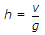# Civil Engineering - Hydraulics

### Exercise :: Hydraulics - Section 1

11.

Most economical section of a circular channel for maximum discharge

 A. depth of water = 0.95 diameter of circular section B. hydraulic mean depth = 0.286 diameter of circular section C. wetted perimeter = 2.6 diameter of circular section D. wetted perimeter = 2.83 depth of water E. all the above.

Explanation:

No answer description available for this question. Let us discuss.

12.

Unit of kinematic viscosity is

 A. m2/sec B. Newton sec/m2 C. Newton sec/m3 D. Kg sec/m2.

Explanation:

No answer description available for this question. Let us discuss.

13.

The moment of inertia of a floating body along its longitudinal axis and the volume of water displaced by it are I and V respectively. The height of the metacentre above centre of buoyancy of the body, is

 A.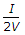B.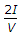C.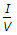D.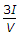E.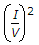Explanation:

No answer description available for this question. Let us discuss.

14.

Time of emptying liquid from a hemispherical vessel through an orifice at its bottom, is

 A.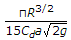B.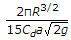C.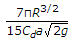D.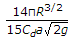Explanation:

No answer description available for this question. Let us discuss.

15.

Critical depth (h) of a channel, is

 A.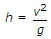B.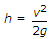C.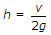D.